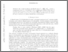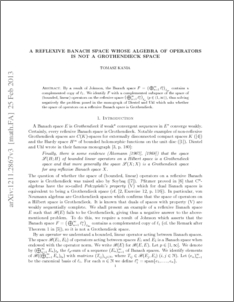# A reflexive Banach space whose algebra of operators is not a Grothendieck space

Kania, Tomasz (2013) A reflexive Banach space whose algebra of operators is not a Grothendieck space. Journal of Mathematical Analysis and Applications, 401 (1). pp. 242-243. ISSN 0022-247XPreview
PDF (1211.2867)
1211.2867.pdf - Submitted Version

By a result of Johnson, the Banach space $F=(\bigoplus_{n=1}^\infty \ell_1^n)_{\ell_\infty}$ contains a complemented copy of $\ell_1$. We identify $F$ with a complemented subspace of the space of (bounded, linear) operators on the reflexive space $(\bigoplus_{n=1}^\infty \ell_1^n)_{\ell_p}$ ($p\in (1,\infty))$, thus giving a negative answer to the problem posed in the monograph of Diestel and Uhl which asks whether the space of operators on a reflexive Banach space is Grothendieck.View Item